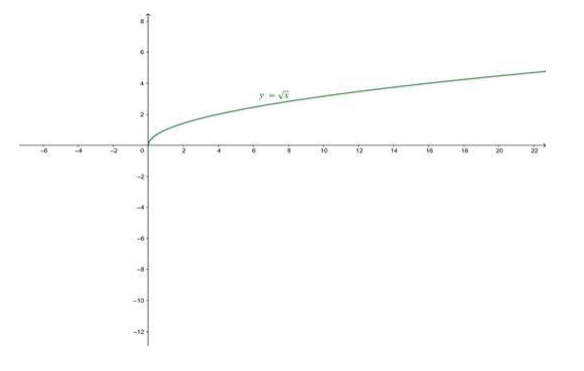# Find the domain and the range of the square root function,

Question:

Find the domain and the range of the square root function,

f: $\mathbf{R}^{+} U\{0\} \rightarrow \mathbf{R} \mathbf{f}(\mathbf{x})=\sqrt{x}$ for all non-negative real numbers. Also, draw its graph.

Solution:

Given

$f(x)=\sqrt{x}$

To Find: Domain and Range of $\mathrm{f}(\mathrm{x})$.

The domain of the given function is set of all positive real

Numbers including 0 . In this case, if the value of $x$ is a negative

The number then it makes the expression undefined.

Therefore,

Domain(f) $=[0, \infty) \forall x \in R^{+} \cup\{0\}$

As the value of $x$ varies from 0 to $\infty$, value of $\sqrt{x}$ varies from $\sqrt{0}$ to $\sqrt{\infty}$, Hence,

Range $(f)=[0, \infty) \forall x \in R^{+} \cup\{0\}$

Graph: